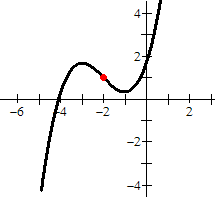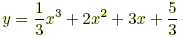index: click on a letter A B C D E F G H I J K L M N O P Q R S T U V W X Y Z A to Z index index: subject areas numbers & symbols sets, logic, proofs geometry algebra trigonometry advanced algebra & pre-calculus calculus advanced topics probability & statistics real world applications multimedia entrieswww.mathwords.com about mathwords website feedback

Point of Symmetry

A special center point for certain kinds of symmetric figures or graphs. If a figure or graph can be rotated 180° about a point P and end up looking identical to the original, then P is a point of symmetry.

 Example:This is a graph of the curvetogether with its point of symmetry (–2, 1). The point of symmetry is marked in red.